#Method of Undetermined Coefficients or Guessing MethodAs for the second order case, we have to satisfy two conditions. One is already satisfied since we assumed that our equation has constant coefficients. The second condition has to do with the nonhomogeneous term g(x). Indeed, in order to use the undetermined coefficients method, g(x) should be one of the elementary forms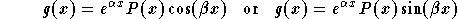,

where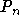is a polynomial function. For a more general case, see the remark below. In order to guess the form of the particular solution we follow these steps:

(1)
Write down the characteristic equation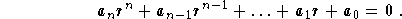Find its roots and (especially) their multiplicity. Note that it will help strongly if you factorize this equation. This way you get the roots and their multiplicity;

(2)
Write down the number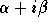(which you generate from g(x)). Then,
(2.1)
ifis not one of the root of the characteristic equation, then set s=0;
(2.2)
ifis one of the root of the characteristic equation, then s is its multiplicity;

(3)
Write down the guessed form for the particular solution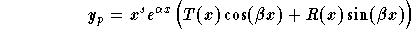,

where T(x) and R(x) are two polynomial functions with degree(T) = degree(R) = degree(P). So, if the degree of P is m, there are 2m+2 coefficients to be determined;

(4)
Plug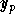into the equation (NH) to determine the coefficients of T and R;
(5)
Write down your final answer for.

Remark: The undetermined coefficients method can still be used if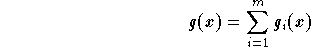,

where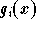has the elementary form described above. Indeed, we need (as we did for the second order case) to split the equation (NH) into m equations. Find the particular solution to each one, then add them to generate the particular solution of the original equation.

Example: Find a particular solution of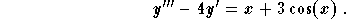Solution: Let us follow these steps:

(1)
Characteristic equation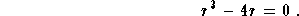We have the factorization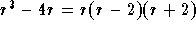. Therefore, the roots are 0,2,-2 and they are all simple.

(2)
We have to split the equation into the following two equations: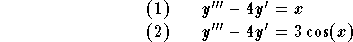;

(3)
The particular solution to the equation (1):
(3.1)
We have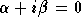which is a simple root. Then s = 1;
(3.2)
The guessed form for the particular solution is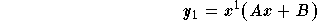,

where A and B are to be determined. We will omit the detail of the calculations. We get A = -1/8 and B=0. Therefore, we have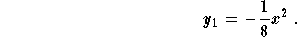;

(4)
The particular solution to the equation (2):
(4.1)
We have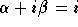which is not a root. Then s = 0;
(4.2)
The guessed form for the particular solution is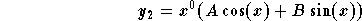where A and B are to be determined. We will omit the detail of the calculations. We get A = 0 and B=-3/5. Therefore, we have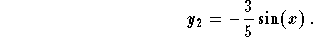;

(5)
The particular solution to the original equation is given by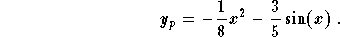[Differential Equations] [First Order D.E.] [Second Order D.E.]
[Geometry] [Algebra] [Trigonometry ]
[Calculus] [Complex Variables] [Matrix Algebra]S.O.S MATHematics home page

Do you need more help? Please post your question on our S.O.S. Mathematics CyberBoard.Author: Mohamed Amine Khamsi